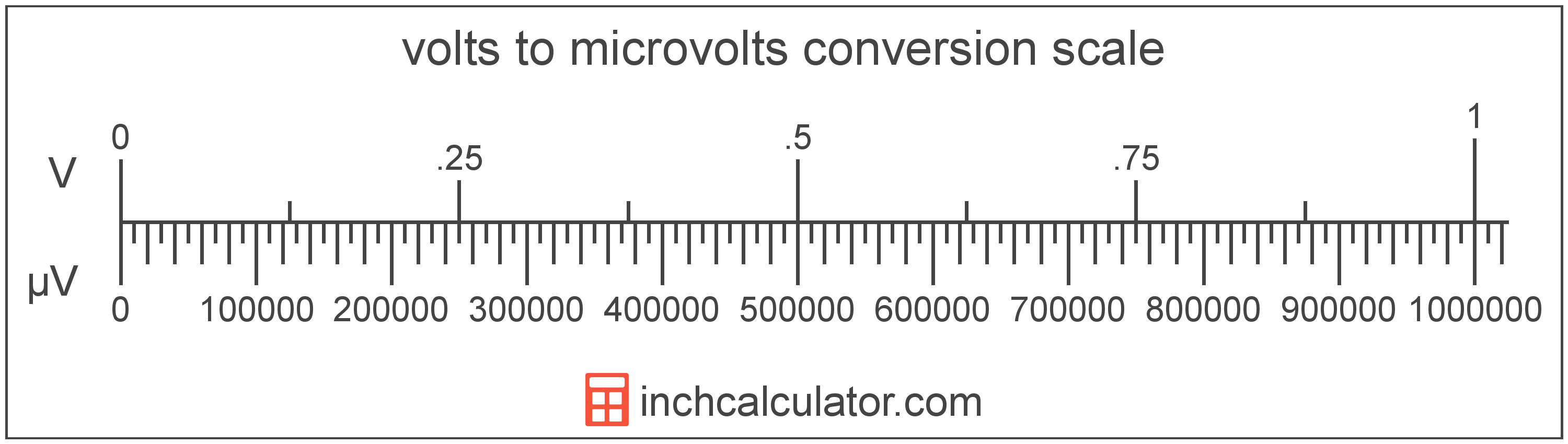# Microvolts to Volts Converter

Enter the voltage in microvolts below to get the value converted to volts.

Results in Volts:1 µV = 1.0E-6 V
Hint: use a scientific notation calculator to convert E notation to decimal

Do you want to convert volts to microvolts?

## How to Convert Microvolts to Volts

To convert a measurement in microvolts to a measurement in volts, divide the voltage by the following conversion ratio: 1,000,000 microvolts/volt.

Since one volt is equal to 1,000,000 microvolts, you can use this simple formula to convert:

volts = microvolts ÷ 1,000,000

The voltage in volts is equal to the voltage in microvolts divided by 1,000,000.

For example, here's how to convert 5,000,000 microvolts to volts using the formula above.
volts = (5,000,000 µV ÷ 1,000,000) = 5 V## What is a Microvolt?

One microvolt is equal to 1/1,000,000 of a volt, which is the potential difference that would move one ampere of current against one ohm of resistance.

The microvolt is a multiple of the volt, which is the SI derived unit for voltage. In the metric system, "micro" is the prefix for millionths, or 10-6. Microvolts can be abbreviated as µV; for example, 1 microvolt can be written as 1 µV.

## What is a Volt?

One volt is equal to the potential difference that would move one ampere of current against one ohm of resistance.

The volt is the SI derived unit for voltage in the metric system. Volts can be abbreviated as V; for example, 1 volt can be written as 1 V.

### Voltage and Ohm's Law

Voltage is a measurement of electromotive force and the electrical potential difference between two points of a conductor.

Ohm's Law states the current between two points on a conductor is proportional to the voltage and inversely proportional to the resistance. Using Ohm's Law, it's possible to express the potential difference in volts as an expression using current and resistance.

VV = IA × R

The potential difference in volts is equal to the current in amperes times the resistance in ohms.

## Microvolt to Volt Conversion Table

Table showing various microvolt measurements converted to volts.
Microvolts Volts
1 µV 0.000001 V
2 µV 0.000002 V
3 µV 0.000003 V
4 µV 0.000004 V
5 µV 0.000005 V
6 µV 0.000006 V
7 µV 0.000007 V
8 µV 0.000008 V
9 µV 0.000009 V
10 µV 0.00001 V
100 µV 0.0001 V
1,000 µV 0.001 V
10,000 µV 0.01 V
100,000 µV 0.1 V
1,000,000 µV 1 V

## References

1. International Bureau of Weights and Measures, The International System of Units, 9th Edition, 2019, https://www.bipm.org/documents/20126/41483022/SI-Brochure-9-EN.pdf